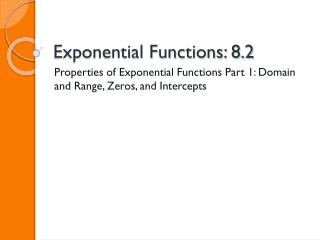# Exponential Functions: 8.2 - PowerPoint PPT PresentationDownload PresentationExponential Functions: 8.2

Exponential Functions: 8.2Download Presentation## Exponential Functions: 8.2

- - - - - - - - - - - - - - - - - - - - - - - - - - - E N D - - - - - - - - - - - - - - - - - - - - - - - - - - -
##### Presentation Transcript

1. Exponential Functions: 8.2 Properties of Exponential Functions Part 1: Domain and Range, Zeros, and Intercepts

2. Exponential Functions: 8.2 Activation: Warm Up pg. 343 B & Motivator Region Tournament-Tutoring/E2020 Quiz #1 B-DAY 2/23 A-DAY 2/24

3. Exponential Functions: 8.2 IMPORTANT DATES QUIZ #1B-DAY 2/23 A-DAY 2/24 MidUnit Test A-DAY 3/5 B-DAY 3/6 Quiz # 2 A-DAY 3/15 B-DAY 3/16 Unit Test B-DAY 3/22 A-DAY 3/23

4. Exponential Functions: 8.2 EQ: What is the basic exponential function? Today we will review how to graph an Exponential Functions!!

5. Exponential Functions: 8.2 • b. Investigate and explain characteristics of exponential functions, including domain and range, asymptotes, zeros, intercepts, intervals of increase and decrease, rates of change, and end behavior.

6. Exponential Functions: 8.2 Review Homework Examples: Pg. 495 (6-9) Pg.496 (13-16)& (25-28) Page p.495/496 #8, 11, 15, 19, 25, 27 Exponential Worksheet #13, 15, & 17

7. Exponential Functions: 8.2 **Remember when you have a negative exponent, you will flip over the fraction far (place the exponent in the opposite segment of the division bar: numerator/denominator) and make the exponent POSITIVE.** **You only flip the variable attached to the exponent; not the coefficient** Examples:

8. Exponential Functions: 8.2

9. Exponential Function: 8.2 Remember??? Basic Linear Function: y= x Basic Quadratic Function: y=x² Fact: Unlike Linear and Quadratic Functions, the basic Exponential Function is not a single function Fact: Exponential Functions depends on the BASE of the Exponential Function

10. Exponential Function: 8.2 The Basic Exponential Function is written as y= for b>0 , b, and x is ANY real number, and b is a positive number Variable x is now the power (exponent), rather than the base like with a linear function Problem #2 page 346 # 1, 3, 4, 5, & 6 Complete Table and Graph Key Terms Review: Zeros (Solutions, X-intercepts)- Set of x-values such that f(x)=0 Ex: x + 3= 0 X =-3 X-intercepts- are the x-coordinate (x-value) of the point where a graph crosses the x-axis. • The values at which the graph crosses the x-axis • To solve set y or f(x) equal to 0 and solve

11. Exponential Function: 8.2 Y-intercept • The y-intercept is the y-coordinate of the point where a graph crosses the y-axis. • The values at which the graph crosses the y-axis • To solve replace x-values with 0 Domain- x-values of a function (-∞, ∞) Range- y-values of a function (0, y- intercept value)

12. Exponential Function: 8.2 Exponential Growth (positive exponent)/Decay (negative exponent or the base is 0 or 1) Problems are examples of Exponential Functions

13. Exponential Function: 8.2 • Exponential functions with a base > 1 (whole #) have the following characteristic: • the higher the number for the base the CLOSER the graph will be to the y-axis/ steeper graph in Quadrant 1 Graph: y= 2ˣ, y= 3ˣ, y=4ˣ, y=5ˣ

14. Exponential Function: 8.2 • Exponential functions with a base between 0 and 1 (fraction) has the following characteristics: • The smaller the fraction or decimal the closer the graph is to the y-axis in Quadrant 2 • The graph falls from left to right (decreases) Graph y= .9ˣ, y= .1ˣ, y= .45ˣ, y=.25ˣ

15. Exponential Function: 8.2 TOTD: 1) What is the difference between an exponential function and a linear/quadratic function?

16. Exponential Function: 8.2 Homework Redo Exponential Properties Review pg. 501-503(6-16)

17. Exponential Function: 8.2 Activation: pg. 343 Warm Up & Motivator Instruction: Notes on Domain, Range, Zeroes, Intercepts Work: Complete Guided Practice Examples-Problem 1 & 2 Assessment: Unit 5 Quiz 1 Summary: What is the difference between an exponential function and a linear/quadratic function?